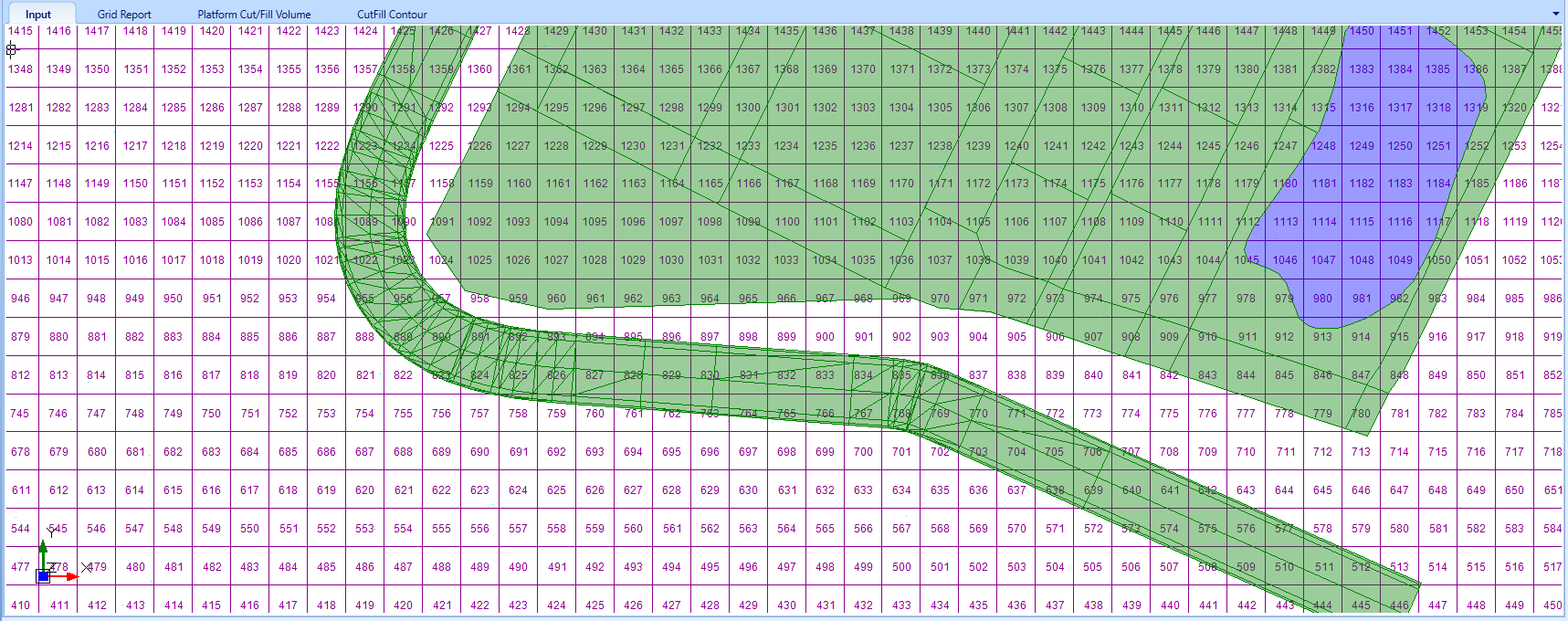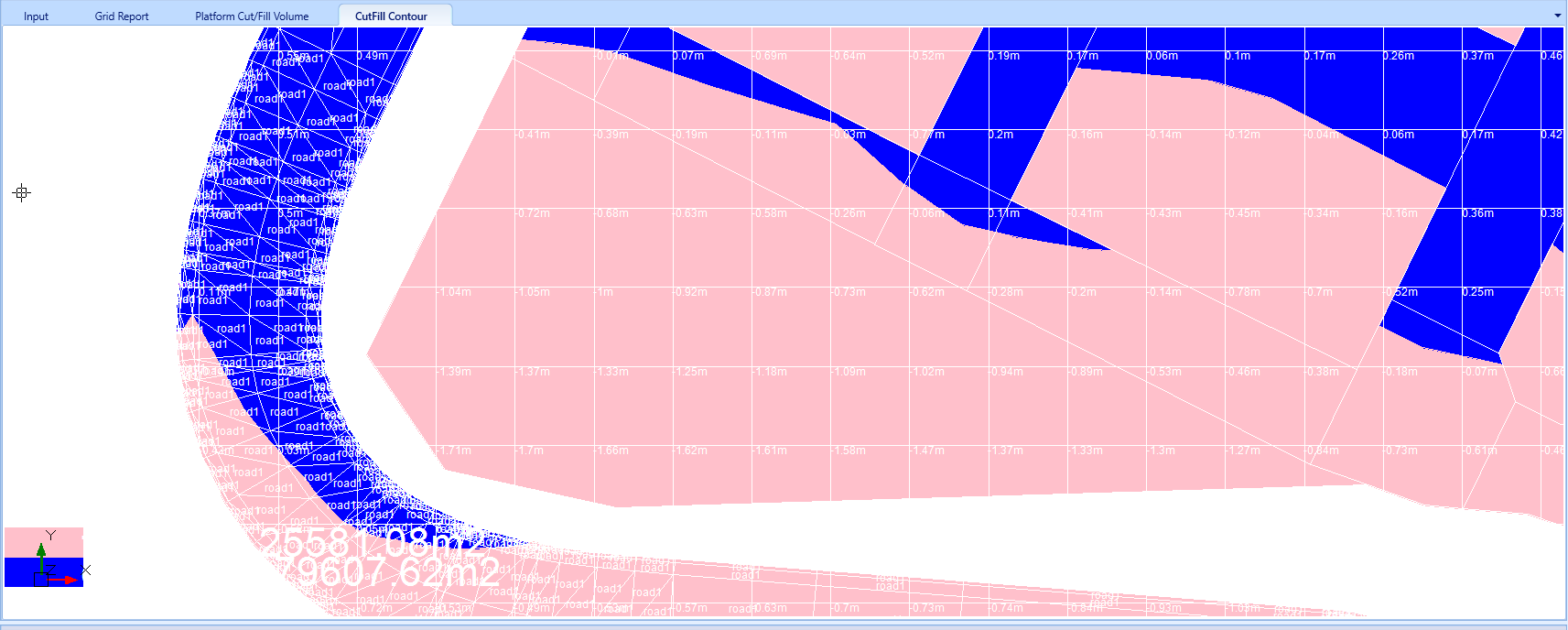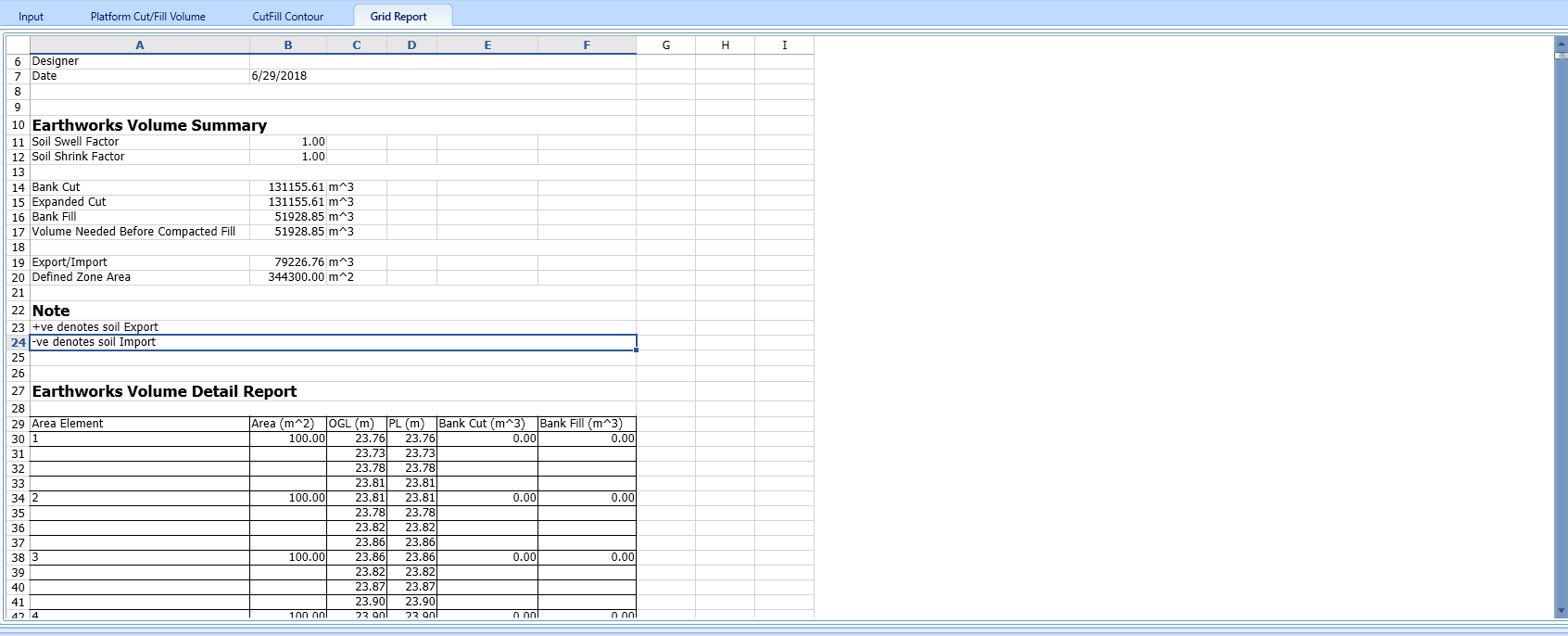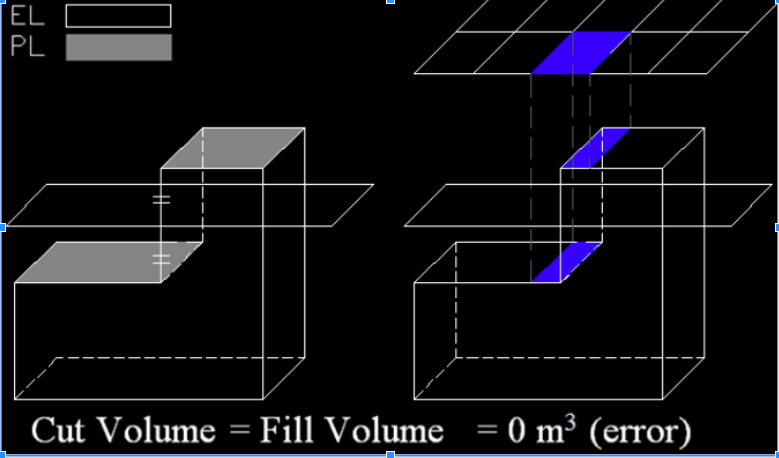603 - 5885 1250 (4 lines) [email protected]
Select Page

GRID METHOD is one of the most popular methods to compute the cut and fill earthworks, due to its simplicity and intuitive of use.

## Methodology:

1. One starts with a predetermined grid size
2. One draws a uniform grid onto the plan of the earthwork projects.3. For each grid point, one reads of the existing ground level and the propose level. And then one takes the average depth of the existing ground level$$GL=GL_1+GL_2+GL_3+GL_4$$ and the proposed level of the four points $$PL=PL_1+PL_2+PL_3+PL_4$$.
4. If the average existing ground level is higher than the proposed level, then it is a cut. Or else it is a fill. In MES software, the differentials of the ground level and proposed level is indicated on the cut/fill contour, as shown below5. For each grid cell $$i$$, the difference between ground level and the proposed level, multiply with the grid area, gives the total grid volume ($$V_i=A_i(GL-PL)$$), which can be tabulated in an Excel report for the ease of checking.6. To obtain the total cut and fill volume, one just needs to sum up the contribution from all of the grids.

1. Easy to use. It’s very intuitive and very easy to understand
2. Easy to check. All you have to do is to just take the elevation average, calculate the volume, and minus the two volumes off each other.

## Drawbacks:

1. Very dependent on the grid size
2. The elevation levels are captured at uniform grid node, which means that at those points, the elevation are derivative of the given surveyor points. This will depends on the interpolation method used, and hence, a loss of accuracy there.
3. Cannot capture the critical inflection lines within a grid cell when the cut/fill condition goes from cut to fill, or vice versa. Below are the two cases where this can occur:Grid method will errorneously report zero contribution for cut and fill volume if both occur in the same cell